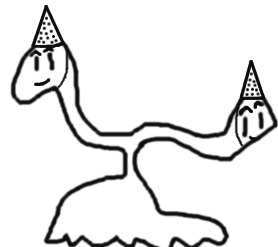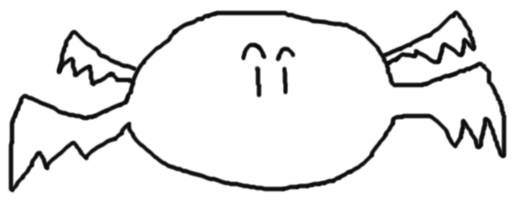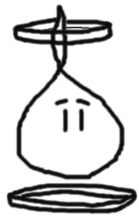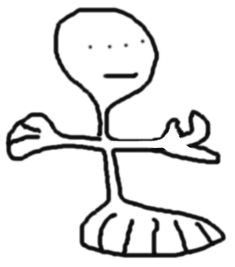# Alien Birthday Paradox

Did you know that if 23 people are chosen at random, the chance of at least two of them having the same birthday is greater than 50%?

This is a very famous result in mathematics known as the Birthday Paradox … however its explanation, despite using simple concepts of probability, is not something very intuitive (I have tried to explain it to several colleagues in mathematics and I think that until today they did not understand why this result).

The first time this paradox is spoken to us, we imagine that in order to have a chance of two or more people having a birthday on the same day greater than 50%, we will need at least 183 people … however it is a very wrong idea. We will do a step by step to understand why with just 23 people it is already possible to reach this probability.

Case 0: We choose only one person. Chance of two or more people having a birthday on the same date, 0%, as there is only one person.

Case 1: We add one person to Case 0. Now the calendar is already filled in (due to Case 0), so the chance of this new person not having a birthday on the same day as another, is 364/365.

Case 2: We add one person to Case 1. Now the calendar is already filled with two spaces (due to Case 1), so the chance of this new person not having a birthday on the same day as another, is 363/365.

Case 3: We add one person to Case 2. Now the calendar is already filled with three spaces (due to Case 2), so the chance of this new person not having a birthday on the same day as another, is 362/365.

Case 4: We add one person to Case 3. Now the calendar is already filled with three spaces (due to Case 3), so the chance of this new person not having a birthday on the same day as another, is 361/365.

However, note that the result of Case 2 depends on the negative result of Case 1. Just as the result of Case 3 depends on the negative result of Case 2 which depends on the negative result of Case 1. Similarly, the result of Case 4 depends on the negative result of Case 3, which depends on the negative result of Case 2, which depends on the negative result of Case 1. So, in order to arrive in the context of Case N, we need the previous N-1 Cases to have obtained a negative result.

Doing the math, we have to:

Case 1: (364/365) = 99,7%

Case 2: (364/365).(363/365) = 99,1%

Case 3: (364/365).(363/365).(362/365) = 98,3%

Case 4: (364/365).(363/365).(362/365).(361/365) = 97,2%

Case 22: (364/365).(363/365)…(345/365).(344/365) = 49,2%

Thus, we arrived at the result that with just 23 people, the chance that none of them will have a birthday on the same date as another, is 49,2%. Reversing the proposition, the chance that two or more people will have birthdays on the same dates is 50,8%.

The result is cool, but there are already many books, blogs and other channels talking about this same concept and showing these calculations, so we will do something more fun. We will calculate for each of the other 7 planets in our Solar System, how many extraterrestrials we need to choose at random for the chance of two of them having a birthday on the same day (relative to that planet) to be greater than 50%.

MERCURY: In the period of translation of Mercury around the Sun, the planet rotates and a half around its own axis … that is, there is only one complete day for each Mercurian Year. That way, all Mercurians have a birthday on the same day.VENUS: In the period of translation of Venus around the Sun, the planet does not complete a complete turn around its own axis … that is, the days of Venusians are greater than their years. That is, there are no birthdays on Venus.MARS: In the period of translation of Mars around the Sun, the planet makes 669 turns around its own axis… that is, there are 669 days in each Martian year. By repeating the calculation of the birthday problem, we find that if we randomly gather 31 Martians, there is a 50.5% chance that at least two of them have birthdays on the same date (considering the Martian calendar).JUPITER: In the period of Jupiter’s translation around the Sun, the planet makes 1.768 turns around its own axis … that is, there are 1.768 days in each Jupiterian year. Repeating the calculation of the birthday problem, we found that if we randomly gather 50 Jupiterians, there is a 50.3% chance that at least two of them will have a birthday on the same date (considering the Jupiterian calendar).SATURN: In the period of Saturn’s translation around the Sun, the planet makes 24.437 turns around its own axis … that is, there are 24.437 days in each Saturnian year. Repeating the calculation of the birthday problem, we found that if we randomly gather 113 Saturnites, there is a 50,3% chance that at least two of them will have a birthday on the same date (considering the Saturnian calendar).URANO: In the period of Uranus translation around the Sun, the planet makes 42.875 turns around its own axis … that is, there are 42.875 days in each Uranian year. Repeating the calculation of the birthday problem, we found that if we gather 245 Ukrainians randomly, there is a 50,2% chance that at least two of them will have a birthday on the same date (considering the Ukrainian calendar).NEPTUNE: In the period of Neptune’s translation around the Sun, the planet makes 89.661 turns around its own axis … that is, there are 89.661 days in each Neptunian year. Repeating the calculation of the birthday problem, we found that if we gather 353 Neptunians at random, there is a 50,0% chance that at least two of them will have a birthday on the same date (considering the Neptunian calendar).